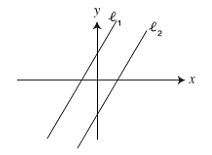Question 9 Selected one answer choice

5000

The equation of l1 is y=2x+ 6. If l2 is parallel to A. which of the following could be the equation of l2?A.y=-4x+2

B.y=-2x+4

C.y=2x-4

D.y=2x+4

E.y=4x+2